You can use the Gravity PropertyManager to apply gravity loads to a part or assembly document for use in structural and nonlinear analyses.

1. Do one of the following:
• In the Simulation study tree, right-click External Loads and select Gravity.
• Click Simulation > Loads/Fixture > Gravity.
• Click Gravity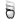(Simulation toolbar).
2. In the PropertyManager, select a planar face, a reference plane, or a straight edge.
The selected reference appears in the Face, Edge, Plane for Direction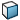box under Selected Reference.
3. Enter a value of gravity or click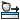to apply Earth's gravity. Select Reverse direction if required. The direction of applied gravity is shown by an arrow in the graphics window. The gravity is applied normal to selected plane or face and along a selected edge.
4. Under Advanced, do the following:
1. Select the desired system of Unit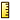you want to use to enter the acceleration values.
2. Enter values for the acceleration components.
If you select a reference plane or a planar face, specify at least one of the following:
• Along plane Dir 1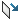. Type the value of the acceleration component along direction 1 of the selected plane or face.
• Along plane Dir 2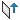. Type the value of the acceleration component along direction 2 of the selected plane or face.
In an assembly, you can use reference geometry from the assembly or the components (parts and subassemblies) of the assembly.
5. For nonlinear studies, you can define a time-dependent gravitational acceleration.
1. Under Variation with Time choose one of the following:
• Linear. To use a default linear time curve which passes through the points (0,0) and (tend, Ai). Where Ai is the value of the i-component of the acceleration specified in the Gravitational Acceleration box and tend is the End time specified on the Solution tab of the Nonlinear dialog box.
• Curve. To define your own time curve. Click Edit to define or import a time curve. An acceleration component is calculated at any time by multiplying the value specified above by the Y value of the time curve.
2. Select Graph to display the actual time-dependent acceleration component(s).
6. If necessary, under Symbol Settings, do the following:
1. Click Edit color to change the color of the gravitational acceleration symbols.
2. Click the spin arrows to increase or decrease the size of the gravitational acceleration symbols.
7. Click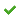.